Home > CCA2 > Chapter 11 > Lesson 11.1.3 > Problem11-33

11-33.
1. What do you notice about the two problems below when you simplify them? Homework Help ✎

1.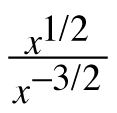2.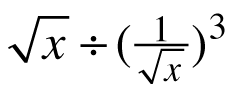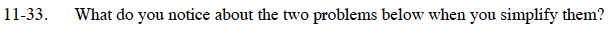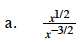Use properties of exponents to simplify.

$\small x^{\frac{1}{2}} \cdot x^{\frac{3}{2}}$

$\small x^{\frac{4}{2}}$

x2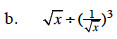$\small x^{\frac{1}{2}}\div\left( x^{-\frac{1}{2}} \right)^3$

$\small \frac{x^{\frac{1}{2}}}{x^{-\frac{3}{2}}}$

See part (a).